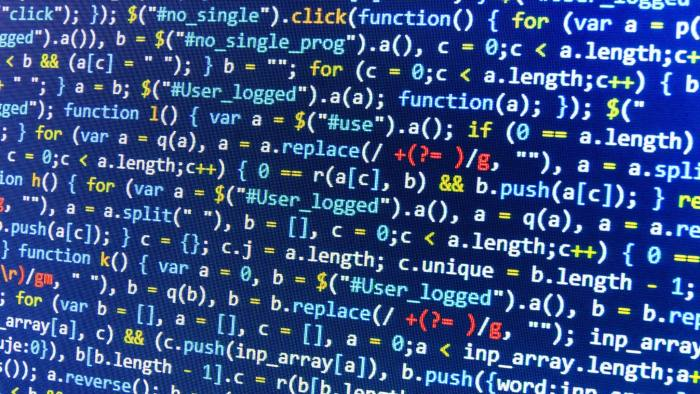### CSCI 3104: Algorithms

#### Course Objectives

In this course, students will

• become familiar with "standard'' algorithms for abstract problem solving;
• learn how to mathematically prove properties of algorithms, including their correctness;
• analyze the time and space complexity of algorithms
• understand the relative merits or demerits of different algorithms in practice
• adapt and combine algorithms to solve problems that may arise in practice
• learn common strategies in the design of new algorithms for emerging applications

#### Topics Covered

Roughly, we will cover the following topics (some of them may be skipped depending on the time available).

• Introduction to Algorithms: Complexity analysis
• Divide and Conquer Algorithms
• Sorting and Order Statistics
• Greedy Algorithms
• Hash functions and Probabilistic Analysis
• Dynamic Programming
• Graph Algorithms: Search, Minimum Spanning Trees, Shortest Paths, Network Flows
• Matching algorithms
• Basic Computational Complexity: P, NP, reductions and open problems